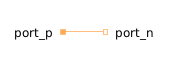Variance - MapleSim Help

Variance

Calculates the empirical variance of its input signalDescription The Variance component calculates the empirical variance of its input signal. The parameter ${t}_{\mathrm{\epsilon }}$ is used to guard against division by zero (the variance computation starts at ${t}_{0}+{t}_{\mathrm{\epsilon }}$ and before that time instant $y=0$). The variance of a signal is also equal to its mean power.Equations $y=\left\{\begin{array}{cc}\mathrm{max}\left(0,\mathrm{var}\right)& {t}_{0}+{t}_{\mathrm{\epsilon }}\le t\\ 0& \mathrm{otherwise}\end{array}$ $\frac{d\mathrm{\mu }}{\mathrm{dt}}=\phantom{\rule[-0.0ex]{0.5ex}{0.0ex}}\left\{\begin{array}{cc}\frac{u-\mathrm{\mu }}{t-{t}_{0}}& {t}_{0}+{t}_{\mathrm{\epsilon }}\le t\\ 0& \mathrm{otherwise}\end{array}$ $\frac{d\mathrm{var}}{\mathrm{dt}}=\left\{\begin{array}{cc}\frac{{\left(u-\mathrm{\mu }\right)}^{2}-\mathrm{var}}{t-{t}_{0}}& {t}_{0}+{t}_{\mathrm{\epsilon }}\le t\\ 0& \mathrm{otherwise}\end{array}$Connections

 Name Description Modelica ID $u$ Noisy input signal u $y$ Variance of the input signal yParameters

 Name Default Units Description Modelica ID ${t}_{\mathrm{\epsilon }}$ $1·{10}^{-7}$ $s$ Variance calculation starts at ${t}_{0}+{t}_{\mathrm{\epsilon }}$ t_epsModelica Standard Library The component described in this topic is from the Modelica Standard Library. To view the original documentation, which includes author and copyright information, click here.